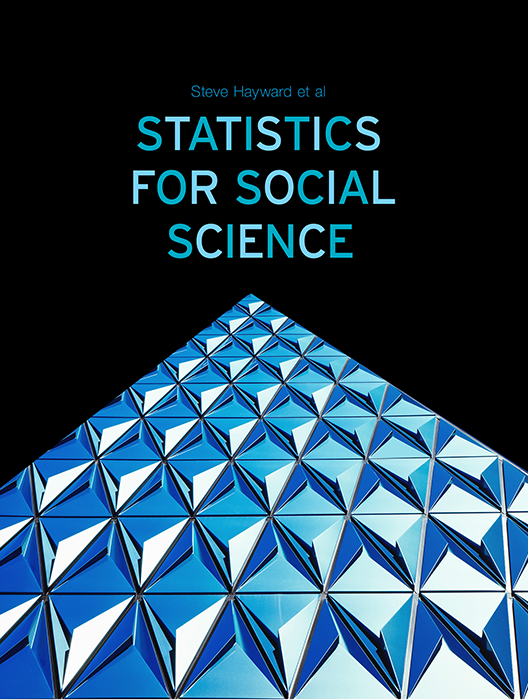# Statistics for Social Science

Lead Author(s): Stephen Hayward

Student Price: Contact us to learn more

Statistics for Social Science takes a fresh approach to the introductory class. With learning check questions, embedded videos and interactive simulations, students engage in active learning as they read. An emphasis on real-world and academic applications help ground the concepts presented. Designed for students taking an introductory statistics course in psychology, sociology or any other social science discipline.

This content has been used by 8,525 students

## What is a Top Hat Textbook?

Top Hat has reimagined the textbook – one that is designed to improve student readership through interactivity, is updated by a community of collaborating professors with the newest information, and accessed online from anywhere, at anytime.

• Top Hat Textbooks are built full of embedded videos, interactive timelines, charts, graphs, and video lessons from the authors themselves
• High-quality and affordable, at a significant fraction in cost vs traditional publisher textbooks

## Key features in this textbookOur Statistics for Social Science textbook allows students to manipulate data, visualize the effects discussed, and explore Lightboard videos that feature instructor explanations to reinforce concepts and calculations.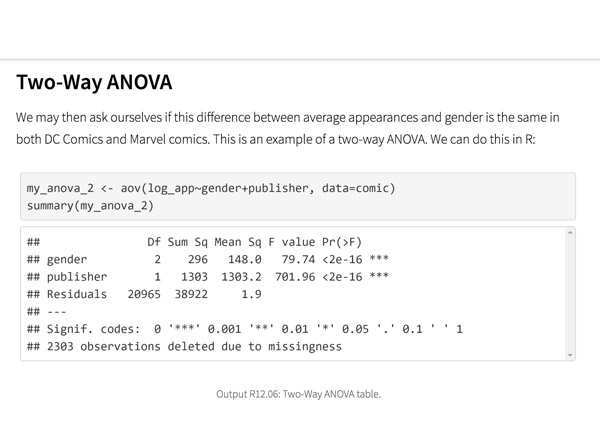Top Hat’s interactive offering includes a complementary module on using R software for data management, graphics, and conducting statistical analyses with examples and practice questions.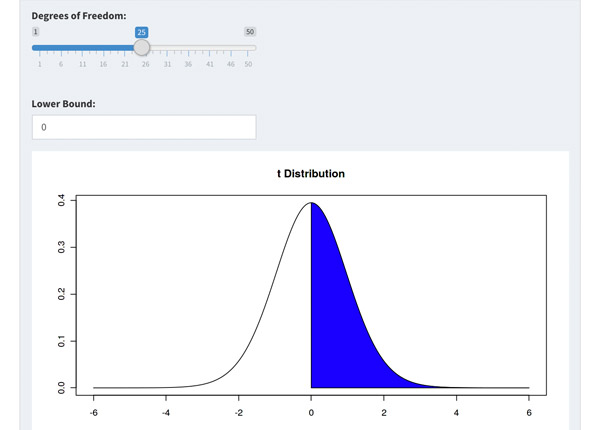Built-in assessment questions embedded throughout chapters so students can read a little, do a little, and test themselves to see what they know!

## Comparison of Social Sciences Textbooks

Consider adding Top Hat’s Statistics for Social Sciences textbook to your upcoming course. We’ve put together a textbook comparison to make it easy for you in your upcoming evaluation.

### Top Hat

Steve Hayward et al., Statistics for Social Sciences, Only one edition needed

### Pearson

Agresti, Statistical Methods for the Social Sciences, 5th Edition

### Cengage

Gravetter et al., Essentials of Statistics for The Behavioral Sciences, 9th Edition

### Sage

Gregory Privitera, Essentials Statistics for the Behavioral Sciences, 2nd Edition

### Pricing

Average price of textbook across most common format

#### Up to40-60%more affordable

Lifetime access on any device

#### $200.83 Hardcover print text only ####$239.95

Hardcover print text only

#### $92 Hardcover print text only ### Always up-to-date content, constantly revised by community of professors Content meets standard for Introduction to Anatomy & Physiology course, and is updated with the latest content ### In-Book Interactivity Includes embedded multi-media files and integrated software to enhance visual presentation of concepts directly in textbook Only available with supplementary resources at additional cost Only available with supplementary resources at additional cost Only available with supplementary resources at additional cost ### Customizable Ability to revise, adjust and adapt content to meet needs of course and instructor ### All-in-one Platform Access to additional questions, test banks, and slides available within one platform ## Pricing Average price of textbook across most common format ### Top Hat Steve Hayward et al., Statistics for Social Sciences, Only one edition needed #### Up to40-60%more affordable Lifetime access on any device ### Pearson Agresti, Statistical Methods for the Social Sciences, 5th Edition ####$200.83

Hardcover print text only

### Pearson

Gravetter et al., Essentials of Statistics for The Behavioral Sciences, 9th Edition

#### $239.95 Hardcover print text only ### Sage McConnell, Brue, Flynn, Principles of Microeconomics, 7th Edition ####$92

Hardcover print text only

## Always up-to-date content, constantly revised by community of professors

Constantly revised and updated by a community of professors with the latest content

### Top Hat

Steve Hayward et al., Statistics for Social Sciences, Only one edition needed

### Pearson

Agresti, Statistical Methods for the Social Sciences, 5th Edition

### Pearson

Gravetter et al., Essentials of Statistics for The Behavioral Sciences, 9th Edition

### Sage

Gregory Privitera, Essentials Statistics for the Behavioral Sciences, 2nd Edition

## In-book Interactivity

Includes embedded multi-media files and integrated software to enhance visual presentation of concepts directly in textbook

### Top Hat

Steve Hayward et al., Statistics for Social Sciences, Only one edition needed

### Pearson

Agresti, Statistical Methods for the Social Sciences, 5th Edition

### Pearson

Gravetter et al., Essentials of Statistics for The Behavioral Sciences, 9th Edition

### Sage

Gregory Privitera, Essentials Statistics for the Behavioral Sciences, 2nd Edition

## Customizable

Ability to revise, adjust and adapt content to meet needs of course and instructor

### Top Hat

Steve Hayward et al., Statistics for Social Sciences, Only one edition needed

### Pearson

Agresti, Statistical Methods for the Social Sciences, 5th Edition

### Pearson

Gravetter et al., Essentials of Statistics for The Behavioral Sciences, 9th Edition

### Sage

Gregory Privitera, Essentials Statistics for the Behavioral Sciences, 2nd Edition

## All-in-one Platform

Access to additional questions, test banks, and slides available within one platform

### Top Hat

Steve Hayward et al., Statistics for Social Sciences, Only one edition needed

### Pearson

Agresti, Statistical Methods for the Social Sciences, 5th Edition

### Pearson

Gravetter et al., Essentials of Statistics for The Behavioral Sciences, 9th Edition

### Sage

Gregory Privitera, Essentials Statistics for the Behavioral Sciences, 2nd Edition

## About this textbook

### Lead Authors

#### Steve HaywardRio Salado College

A lifelong learner, Steve focused on statistics and research methodology during his graduate training at the University of New Mexico. He later founded and served as CEO of Center for Performance Technology, providing instructional design and training development support to larger client organizations throughout the United States. Steve is presently lead faculty member for statistics at Rio Salado College in Tempe, Arizona.

#### Joseph F. Crivello, PhDUniversity of Connecticut

Joseph Crivello has taught Anatomy & Physiology for over 34 years, and is currently a Teaching Fellow and Premedical Advisor of the HMMI/Hemsley Summer Teaching Institute.

## Explore this textbook

Read the fully unlocked textbook below, and if you’re interested in learning more, get in touch to see how you can use this textbook in your course today.

# Four DistributionsVisualization of Rayleigh-Taylor Instability Problem and the distribution of fluids. 

## Chapter Objectives

After completing this chapter, you will be able to:

• Identify features of normal distributions, t-distributions, chi-square distributions, and F-distributions
• Compare the four major distributions in terms of their characteristics
• Identify critical values of z, t, χ2, and F
• Associate probabilities with critical values from tables
• Explain the relationship between degrees of freedom and distribution shape

## Introduction

So far we’ve focused mainly on the normal distribution and its graph, the normal curve. The normal distribution is useful because it can be used to represent the way frequencies that are associated with so many naturally occurring events tend to be distributed.

As it turns out, there are other distributions that are also useful in the process of statistical decision making. We’ll introduce three more of those distributions here, and you’ll see more about them in succeeding chapters. The four distributions that you’ll learn to use in this course are:

• The normal distribution
• The t-distribution
• The chi-square distribution
• The F-distribution

All of the statistical techniques that you will learn in this course will involve using one of these distributions. There are additional distributions that researchers sometimes use, but in-depth coverage of those is beyond the scope of this introductory course.

## Early HistoryFigure 7.1: Galileo Galilei​ ​

Galileo Galilei noted in the 17th century that errors in measurement by astronomers were symmetric and that small errors occurred with greater frequency than large errors.

Carl Friedrich Gauss, a mathematician in the early 19th century, developed the formula for the normal distribution and demonstrated that errors in measurement fit this distribution.

Pierre-Simon Laplace, a few years later, showed that even if the underlying distribution is not normally distributed, the means of repeated samples from the distribution would be very nearly normally distributed and that the larger the sample size, the more closely the distribution of means approximated a normal distribution. This, in turn, led to the discovery of the Central Limit Theorem.Figure 7.2: Abraham de Moivre​​ ​

Abraham de Moivre, who at that time was a statistician and a consultant to gamblers, made lengthy computations of the outcome of events and noted that as the number of binomial events, such as coin flips, increased, the shape of that distribution approached a very smooth curve approximating the normal distribution.

## The Normal Distribution

You learned in the Normal Distribution chapter that most distributions of data in nature are said to be normally distributed, meaning that when graphed they tend to be unimodal and symmetrical and appear as a bell-shaped distribution, like the distribution in Figure 7.3 below.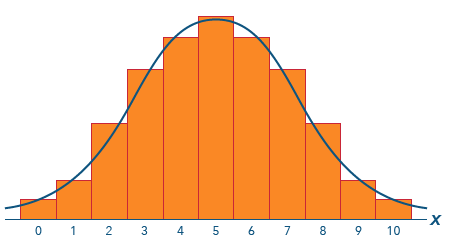Figure 7.3: A normal curve with histogram​

In a normal distribution, most scores are clustered around the middle of the distribution, with fewer scores out towards the tails. This also indicates the relative probability of selecting a given score by chance (i.e., if we were to draw a score at random from the distribution, we would be more likely to get a score closer to the middle, or mean, than a score closer to the tails, where there are fewer scores).

### Calculating z-Scores

You also learned that raw scores in a distribution can be converted to z-scores, representing standard deviation units, by dividing the difference between the raw score and the distribution’s mean by the standard deviation. The formula below is used for finding the z-score for a single value.

The result of that is equal to the distance the raw score is from the mean expressed in terms of standard deviations of that data set. The resulting z-score can then be used to compare scores across distributions since the scores are now expressed in common units of z. Converting the raw scores in this manner has the effect of standardizing the scores. The distribution that results from this is a standardized version of the normal distribution called the standard normal distribution.

### Calculating a z-Score for a Sample Mean

Just as a z-score can be used to determine the probability associated with a single value, a slightly different version of the statistic can be used to determine the probability associated with a sample mean. The difference involves substituting the standard error of the means (SEM) for the population standard deviation in the denominator of the formula. It now looks like this:

Recall that while the standard deviation is an individual measure, indicating how far individuals within the same sample vary from the sample mean, the standard error indicates the variability of the distribution of all possible sample means with a particular sample size. The sample standard deviation is divided by the square root of n because as sample size increases, the variability of this sampling distribution about the population mean decreases (i.e., the larger the sample, the more accurate the sample value should become as an estimate of the population value).

Note: z-Scores for sample means are often referred to (and perhaps more appropriately) as z-statistics. You’ll see more about this use in later chapters.

## The Standard Normal Distribution

The graph of the standard normal distribution appears to be very much like any other normal distribution except that it is now composed of z-scores (“standard deviation units”) instead of the original raw score units. Comparing those z-scores is how we’re now able to compare scores across distributions. The standard scores now share a common distribution with a common mean and standard deviation.

The mean of the standard normal distribution is always zero, and the standard deviation is always 1.0. This does two things that benefit researchers:

• Since the mean and standard deviation are now standardized, cross-distribution comparisons of scores can be made.
• Since the distribution is normal, we can make determinations about the probabilities associated with the scores.

We can determine probabilities because we know the proportion of scores included in any given portion of the normal distribution, seen below as standard deviation units, which in turn equal z-score units. We can map these proportions to a representation of the normal curve as shown below.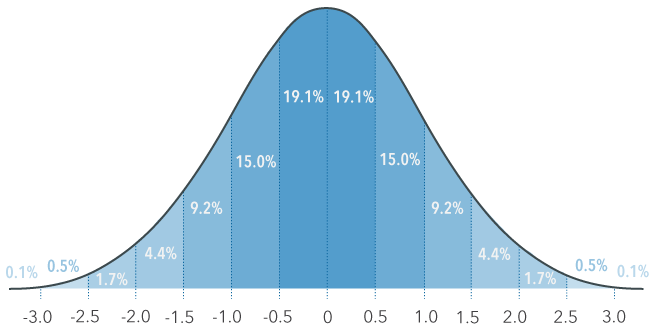Figure 7.4: A normal curve and probability​

We often refer to these as “areas under the curve,” since this is how they are often pictured (see Figure 7.4). More importantly, those areas correspond to proportions, which in turn correspond to probabilities. The rationale is that if a given proportion of scores lie within a certain area, then the probability of randomly selecting a score that falls within that area is equal to that proportion.

We can take this a step further by referring to the Standard Normal Table.

## The Standard Normal Table

The standard normal table, or z-table, enables us to determine probabilities associated with z-scores. There are various versions of the table published, of which Figure 7.6 is one. This version shows areas below z in the lower half of the distribution.

• To get the area above z, subtract the area shown in the table from 1 to get the area to the right of z.
• To get an area in the upper tail, use the area from the equivalent lower tail area and change the sign from minus to plus.
• To get an area between two z-scores, subtract the lower area from the higher area.Figure 7.5: Area to the left of the z-score​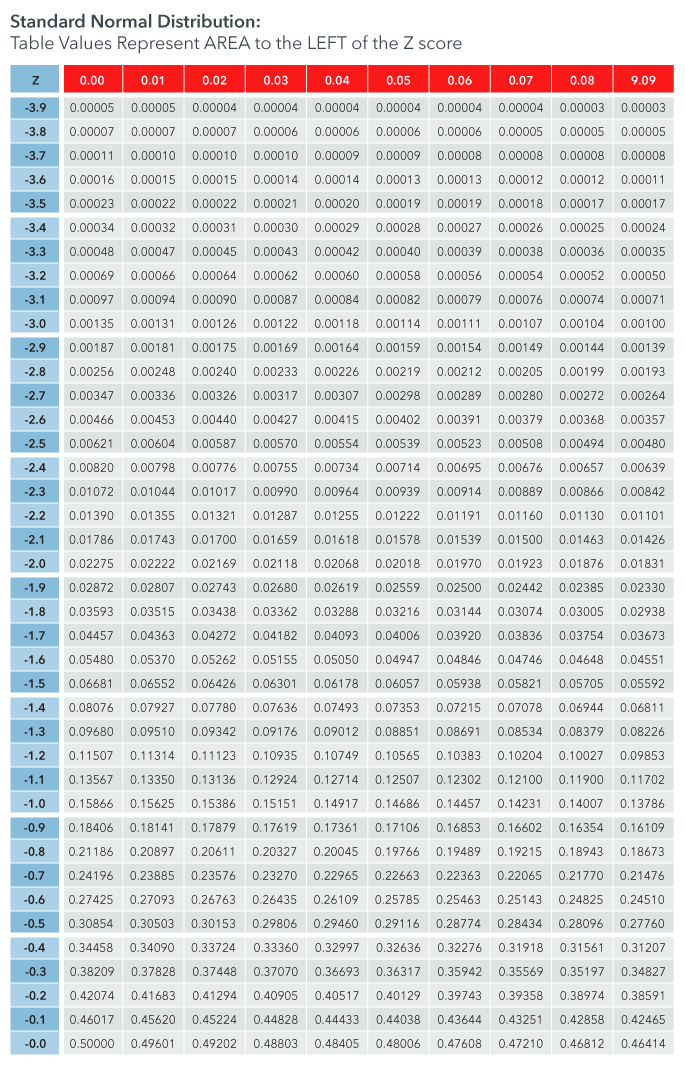Figure 7.6: Standard normal table​​

### Critical Values of z

Critical values refer to values of z that cut off, or act as boundaries for, areas of the distribution that are of particular interest for some reason. You’ll see in the next chapter how they are used to help define confidence intervals and, in later chapters, how they help researchers make decisions about relationships in data they’ve collected. For now, we’ll consider a critical value to be a z-score that can be used to identify a particular area of the distribution.

Researchers often want to be able to determine whether a measure (a score) has a low probability of having occurred by chance, i.e., it may be important to know whether an outcome that has been measured in some way has a low or high probability of being the direct result of something the researcher has done or is likely to be due simply to random chance. Key to making a judgment like this is being able to identify critical values of the distribution that help to determine the probability associated with events.

It’s useful to start with critical values for the standard normal distribution because that distribution is a constant, i.e., there is only one standard normal distribution and it is defined in such a way that the proportions of areas, and therefore the probabilities associated with those areas, remain constant.

Example: z-Score Probability

A school counselor would like to know whether the WAIS (Wechsler Adult Intelligence Scale) IQ score of a gifted student she is counseling is in the upper 5% of the general population. The WAIS has published norms for the general population showing the mean is 100 and the standard deviation is 15. The student in question scored 125 on the test. The counselor used this information to determine that the student’s z-score equivalent was z = 1.67. Here is the calculation:

Using the critical value table above, she then determined that the critical value for z that cuts off the upper 5% of the distribution is 1.64. You can check this by using the value for -1.64 in the table above; since the distribution is symmetrical, the area above +1.64 is the same as the area below -1.64. The area shown, .05050, is the closest area to .05 so the counselor used the corresponding z-score as her critical value. (Note that there can be some argument here; some researchers use 1.65 as the critical value of z marking off 5% of the area of the distribution, while others split the difference and use 1.645. You’ll see more about this in upcoming chapters.)

Since the counselor’s calculated z-score for the student was 1.67 and since that is above the critical value of 1.64 she is able to conclude the student has an IQ score falling in the upper 5% of the general population.

7.01

Which of the following is an important use of the standard normal distribution?

A

Comparing scores within distributions

B

Determining whether scores are uniformly distributed

C

Comparing scores across distributions

D

Determining whether scores are skewed

7.02

Critical values ________ areas of a distribution that are of particular interest.

7.03

What is the area above a z-score of 1.85?

## The t-Distribution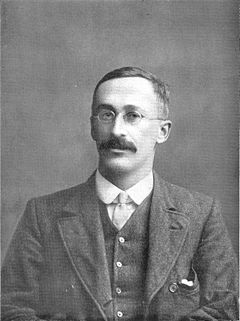Figure 7.7: William Gosset​ 

We credit development of the t-distribution to William Gosset, a statistician employed by Guinness Brewery in Dublin. His work involved testing characteristics of samples of raw materials used in the production of beer. Many of those samples were small, often less than a half dozen or so, and he found the normal distribution did not work well in these conditions. Gosset developed the t-distribution in response to this need and published a paper in 1908 in Biometrika where he referred to the distribution as the “frequency distribution of standard deviations of samples drawn from a normal population.”Figure 7.8: Sir Ronald Fisher​ 

Gosset’s original paper was published under the pseudonym “Student,” as Guinness at the time did not want the association with the company’s brewing processes to be public knowledge. The distribution has since often been referred to as Student’s-distribution. It was publicized largely through the work of the English statistician and biologist, Sir Ronald Fisher, known as the father of modern statistics and experimental design.

The t-distribution is actually an entire family of distributions, unlike the standard normal distribution, which is a single distribution. Like the standard normal distribution, the various t-distributions are unimodal and symmetrical about the mean. The t-distributions, however, tend to be flatter and with thicker tails than the normal distribution.

The various t-distributions are identified by the degrees of freedom associated with them. The degrees of freedom are in turn determined by the sample size n, as you’ll see below.Figure 7.9: Chart of t-distribution for various degrees of freedom​

### Properties of the t-Distribution

• The t-distribution is unimodal and symmetrical about the mean.
• It has µ = 0 and σ > 1 (although σ becomes close to 1 with many df).
• The value of the t-statistic ranges from -∞ to +∞.
• It shares the bell curve of the z-distribution but reflects the variability associated with smaller sample sizes.
• The shape is dependent on the sample size n.
• As the sample size increases, the shape approaches that of the standard normal distribution.
• When df = ∞ the t-distribution exactly matches the standard normal distribution (z).

### Degrees of Freedom and Variance

The rationale for degrees of freedom, expressed as df, is that in order to calculate the variance of a random sample, we must first calculate the mean of that sample and then compute the sum of the squared deviations from that mean. While there will be n such squared deviations, only (n - 1) of them are, in fact, free to assume any value. This is because the final squared deviation from the mean must include the one value of x such that the sum of all the x’s divided by n will equal the mean of the sample. All of the other (n - 1) squared deviations from the mean can, theoretically, take on any values whatsoever, i.e., they are free to vary. For these reasons, the statistic s² is said to have only (n - 1) degrees of freedom.

### The t-Distribution and Sample Size

As sample size increases, the t-distribution becomes more and more like the normal distribution. This can be clearly seen in Figure 7.9 above. When n is greater than or equal to 30, the sampling distribution so closely approximates the normal distribution that, for all practical purposes, the normal distribution can be used instead. This is due to a statistical property called the central limit theorem. Thus, when making statistical comparisons involving samples, t-statistics are used for comparisons when n < 30, and z-statistics can be used when n ≥ 30.

Note: Many statistical software packages use t regardless of sample size, which leads to the same outcome.

## Monte Carlo Experiments

You might wonder how we know that the t-distribution curve tends to flatten out and become more spread out as the sample size decreases. Well, thankfully, statisticians and other mathematicians conduct experiments known collectively as Monte Carlo experiments. In a Monte Carlo experiment, random samples of varying sample size are repeatedly drawn from a large population. Statisticians then study how well the sample data approximate the population data. It turns out that one factor that really impacts the shape of the distribution is sample size.

You can learn more about Monte Carlo experiments here.This Content is Locked
Only a limited preview of this text is available. You'll need to sign up to Top Hat, and be a verified professor to have full access to view and teach with the content.

### Calculating a t-Statistic

A t-statistic, like a z-score or z-statistic, is a standardized test statistic. It enables you to take a score from a sample and transform it into a standardized form very similar to a z-score. To compute a t-statistic, we still need to know the mean of our sample but we can use the standard deviation of the sample as an estimate of the standard deviation of the population. This enables us to compute the statistic when we are working with a small sample and don’t know the standard deviation of the population involved, which, as it turns out, is most of the time.

To calculate a t-statistic for a sample, use the formula below. This formula is very similar to the formula for calculating a z-statistic for a sample. It uses the standard error of the mean σ in the denominator. The difference between the z-statistic and t-statistic formulas is just that s is substituted for σ.

### Critical values of t-distributions

The t-distributions are quite similar to the standard normal curve, or z-score distribution. Whereas the z-score curve is fixed, with a standard shape, area, and critical values (e.g. ±1.96 cuts off 2.5% of the area on each end of the curve), the t-distributions are a family of distributions. Each t-distribution has a normal curve-like appearance except that the shape of the curve changes with the sample size. The curve tends to flatten out and become wider along the x-axis as the sample size decreases.

When the sample size is large, the t-distribution and z-distribution are close to identical (and become identical when the df reaches infinity). However, as the sample size decreases and the curve flattens out, the z-scores that would normally be used to determine specific proportions of the area under the curve no longer ‘fit’ the new shape.

In the figure below, the turquoise line denotes the standard-normal curve or z-score distribution. The blue line denotes the representative normal curve when the sample size is 7 (df = 6). The red line denotes the representative normal curve when the sample size is 4 (df = 3).Figure 7.10: Critical values of z and t

Note how, when the sample size is small, the curve appears as if the top of the curve is getting pushed down and the sides are getting pushed out. The curve becomes flatter and the tails become thicker. The numbers in the figure above on the x-axis denote the z-score or t-score beyond which 2.5% of the area below the curve lies, which also gives us the probability of a score falling into that area. The z-score critical value that cuts off the extreme 2.5% of the area (or scores) in both tails is always ±1.96 because the standard normal distribution is a constant. However, the t-score critical value will vary depending on the sample size and associated df. Look again at the figure above. If the red curve was an accurate representation of a data set (sample size = 4; df = 3), using the z-score cut-off of +1.96 would result in cutting off a much larger area than 2.5%. In fact, the area beyond a t-score of +1.96 in the red distribution would actually be more like 9%. Since the critical value of t in this case is not fixed at 1.96, we will need to use a t-table in order to look up the critical value of t.

## The t-Table

Just as the standard normal table, or z-table, enables us to determine probabilities associated with z-scores, a t-table enables us to determine probabilities associated with t-scores. The chief difference is that, while the z-table includes the probability values associated with the z-scores, the t-table includes critical values rather than probabilities. The table is configured in this way because, since each t-distribution is unique as determined by the degrees of freedom associated with it, to show all of the probability values would require a separate table for each possible df value. That would be really hard to deal with! The compromise is to show critical values according to df and critical areas of the distribution cut off by those critical values.

In the table below, the df are given in the left column while the critical values of t are given in the table columns under the probability values. Each probability value in the top header row represents the probability that a score associated with a t-score given in the table would fall into the upper tail of the distribution (blue in the diagram). The confidence level C referred to at the bottom of the table represents the proportion of scores that would fall between positive and negative critical values; you’ll see more about this use of the table in the next chapter.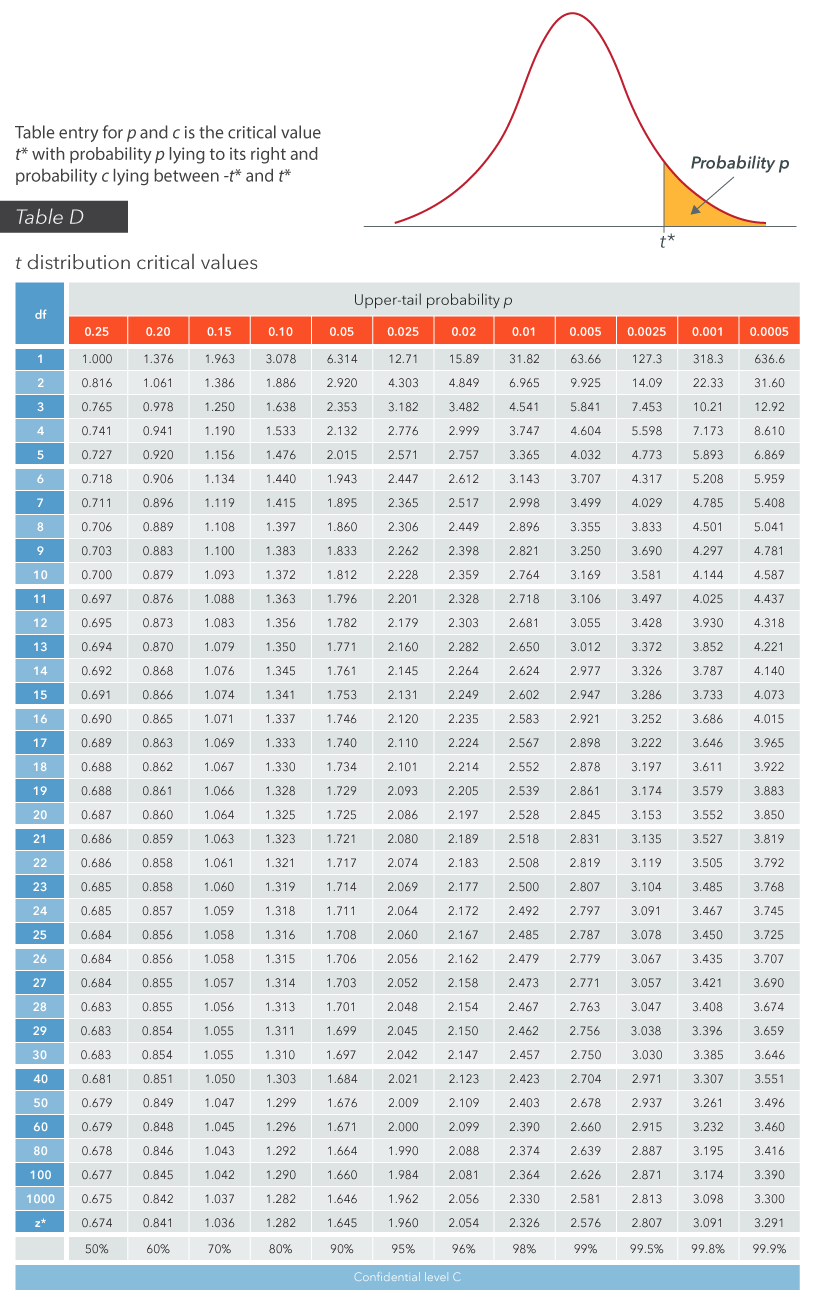Figure 7.11: t-table​

### t-Distribution Demonstration

Use the controls to adjust the degrees of freedom and the upper and lower bounds of the shaded areas to see how the shape changes as a function of df and the areas under the curve accommodate changes in the boundaries.This Content is Locked
Only a limited preview of this text is available. You'll need to sign up to Top Hat, and be a verified professor to have full access to view and teach with the content.

Example: Test Scores

A teacher has determined that a t-statistic based on a sample of 25 achievement test scores with an average score (mean) of 550 and a standard deviation of 75 is t (24) = 3.333. The population mean score is published as 500. She wants to know whether this outcome has a probability of more than or less than .05, or 5%. Here are her calculations:

Checking the critical value table above, she sees that the critical value cutting off 5% of the distribution’s upper tail is t (24) = 1.711. From that, she is able to tell that her outcome would fall in the upper 5% of the distribution.

7.04

A sample that includes scores from eighteen participants would have which of the following?

A

More variability than a sample of 9

B

The same distribution shape as the standard normal distribution

C

Mean, median and mode that are dissimilar.

D

$df$ = 17

7.05

Monte Carlo experiments confirm that ________ affects the shape of a distribution.

7.06

What is the probability of obtaining a t value of 1.860, when df = 8?

## The Chi-Square DistributionsFigure 7.12: Friedrich Helmert​ 

The chi-square distribution derives from early work by the German statistician Friedrich Robert Helmert, who computed the sampling distribution of the sample variance of a normal population. The distribution was independently rediscovered by the English mathematician Karl Pearson and further developed a short time later by Sir Ronald Fisher. It is often referred to as Pearson’s Chi-Squared Test in reference to its use in comparing obtained versus expected proportions of outcomes. You’ll see more about this later when we look at tests for goodness of fit and independence.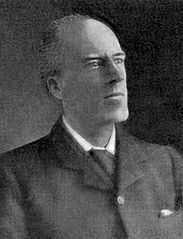Figure 7.13: Karl Pearson​ ​

Like the t-distributions, the chi-square distributions are a family of distributions, each one determined by its degrees of freedom. Unlike both the normal and t-distributions, however, the chi-square distributions are not symmetric but are positively skewed.

The chi-square statistic is represented by the Greek letter chi (χ) squared, indicating it represents values that have been squared. It appears as χ2 and is pronounced “kye.”

### Properties of the Chi-Square Distribution

There are several characteristics of the chi-square distribution that are important to know:

• It is a continuous probability distribution with total area equal to 1.
• The values of the chi-square statistic range from 0 to infinity (∞) with no negative values.
• The distribution is not symmetrical but is skewed to the right (positively skewed).
• The shape depends on the degrees of freedom (df), where df = n - 1 and n equals the sample size (or, as you’ll see, the number of categories).
• The value of the χ2 random variable is always positive.
• There is an infinite number of chi-square distributions.
• As the df increase, the distribution becomes more symmetrical and approaches normalcy.Figure 7.14: Chi-square distributions​​

### Degrees of Freedom and Shape of the Distribution

You can see in the figure above how, with only a few degrees of freedom, the chi-square distribution is severely skewed to the right. As the degrees of freedom (which are directly related to the number of categories of the variable or variables) increase, the shape of the distribution approaches normal and becomes more bell-shaped and symmetrical. With as many as 15 degrees of freedom, the shape of the probability distribution is getting close to normal.

### Calculating Chi-Square

Statistical tests based on chi-square are frequently used to make decisions about population variances and for making decisions about proportions that involve nominal or ordinal level variables. Tests of variances are beyond the scope of this text and will not be presented here.

You will learn to calculate the chi-square statistic when tests for Goodness of Fit and Independence are introduced. For now, it’s enough to know that the statistic is based on comparing differences between observed frequencies and expected frequencies of categorical variables. It enables us to compare how well an observed distribution fits a theoretical distribution. The basic formula is not complex and appears as:

### Critical Values of Chi-Square

Since chi-square distributions are skewed, the critical values cutting off identical areas of the upper and lower tails have different absolute values. The tests that you will learn here, however, only require that you be able to identify critical values in the upper tail of the distribution, thus simplifying the procedure.

These critical values can be determined by using a chi-square table, just as we used z- and t-tables previously.

## The Chi-Square Table

Most versions of the chi-square table present the critical values of chi-square associated with various degrees of freedom and probability levels. They can go on for pages and pages. A relatively simple example of the table looks like Figure 7.15.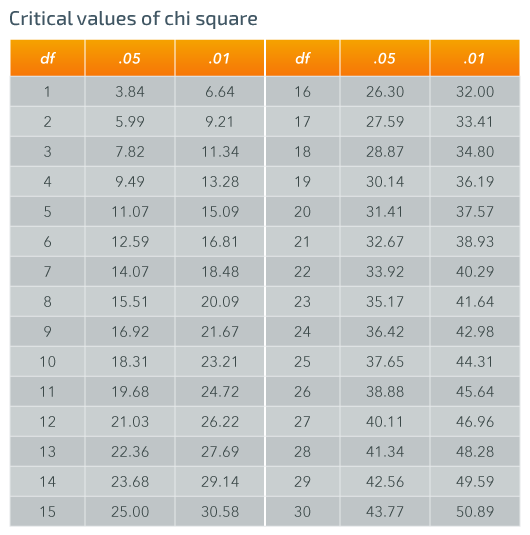Figure 7.15: Chi-square table​

### Using the Chi-Square Table

The columns indicate two levels of probability, .05 and .01. These represent the area of the distribution above the critical value given in the df row. The area equates to the probability of a calculated value of chi-square being equal to or above the critical value.

The use of the table simply involves finding the chi-square value associated with the appropriate number of degrees of freedom (the rows) and the chosen probability level (the columns).

Other versions of this table can be quite comprehensive and include several probability levels and many more rows of degrees of freedom. The table above is sufficient for most chi-square tests, as few chi-square tests involve even as many as 30 degrees of freedom.

Example

A researcher has determined that her calculated chi-square statistic with df = 4 is χ2 = 13.86. She wants to know whether this outcome has a probability of more than or less than .05, or 5%. Checking the table above, she sees that the critical value of chi-square cutting off 5% of the distribution’s upper tail is χ2 (4) = 9.49. From that, she is able to tell that her outcome would fall in the upper 5% of the distribution.

7.07

Which of the following statements about the chi-square distribution is true?

A

Its shape is independent of the degrees of freedom

B

As the $df$ increase, it becomes more symmetric

C

The mean, median, and mode are the same

D

The values of chi-square range from negative infinity to zero

7.08

With few degrees of freedom, the chi-square distribution is severely skewed to the ________.

7.09

With 12 degrees of freedom, click on the critical value of chi-square associated with a probability value of .05.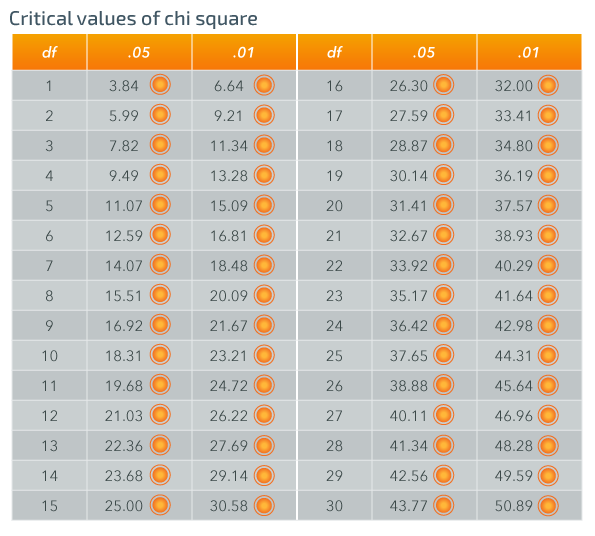## The F-Distribution​

The F-distribution was named in honor of Sir Ronald Fisher, famous for his work in biological statistics and with the t-distribution, but was actually introduced by another statistician, George Snedecor.

The latter was seeking to improve on Fisher’s previously published analysis of variance by incorporating a method of calculating a variance ratio, the result of which he designated by the letter F. Snedecor was a pioneer of modern applied statistics in the U.S. and founded the first academic department of statistics at an American university at Iowa State University.

Like the distributions for z, t, and χ2, the F-distribution is a continuous probability distribution. Like the t-distribution and the χ2 distribution, the F-distribution is really a series of distributions. Like the χ2 distribution, it is also skewed to the right.

### Properties of the F-Distribution

There are several characteristics of the F-distribution that are important to know:

• F-distributions are positively skewed.
• The F-statistic itself is a ratio of two variance estimates.
• The shape of the distribution of F depends on the degrees of freedom associated with the numerator and denominator.
• The total area of an F-distribution (one F-curve) equals 1.
• As the degrees of freedom increase, the distribution becomes less spread out.Figure 7.16: F-Distributions​

### Calculating F

The value of F is calculated as a ratio of two variance estimates, each with its own degrees of freedom. The larger variance is usually the numerator of the ratio; therefore, the value is typically > 1. The df are given as dfN and dfD (sometimes df1 and df2), representing the degrees of freedom for the ratio’s numerator and denominator, respectively.

The method of calculating F given above is used to make comparisons of variances. The rationale is simply that the greater the difference between them, the larger the ratio becomes.

The F-ratio can also be used to compare multiple sample means using what might seem, at first, a rather roundabout method of comparison. As you’ll see, however, the beauty of the method really lies in its inherent simplicity! The comparison still involves a ratio of variances, but instead of comparing sample variances individually, as above, they are combined as below.

Use the sliders in the demonstration below to see how the distribution changes in response to varying df.This Content is Locked
Only a limited preview of this text is available. You'll need to sign up to Top Hat, and be a verified professor to have full access to view and teach with the content.

### Critical Values of F

Since F-distributions are skewed, the critical values cutting off identical areas of the upper and lower tails have different absolute values. The tests that you will learn here, however, only require that you be able to identify critical values in the upper tail of the distribution, thus simplifying the procedure.

These critical values can be determined by using an F-table, just as we used z- and t- and χ2 tables previously.

The values of F are reported as F(dfN,dfD) = (value).

Example

A critical value of F with 4 df in the numerator and 6 df in the denominator with a probability of .05 would be reported as F (4,6) = 4.53.

## The F-Table

The F-table provides critical values for F, just as the other tables you’ve used for z, t, and χ2. There often are separate tables provided for differing probability levels, so the tables may go on for many pages. This is because both the row and column headings in the F-table are used for degrees of freedom since the statistic is a ratio of two values, each with different df.

The table lookup procedure involves finding the appropriate critical values by using the table for the desired probability level and then locating the intersection of the row and column representing the appropriate df values. The dfN are typically shown as column headings with dfD shown as row headings.

Figure 7.17 below is an excerpt from a larger table that combines probability levels with the df headings. It only shows a few degrees of freedom, so in that sense is incomplete, but will serve as an example.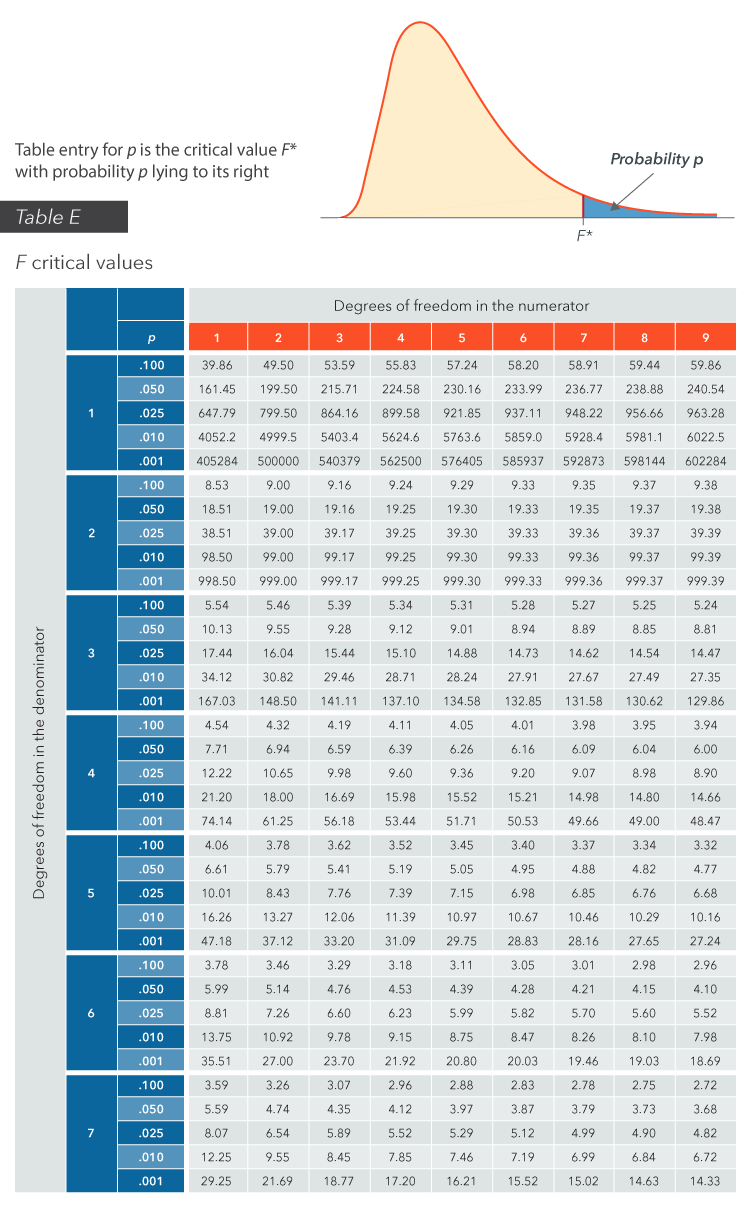​Figure 7.17: F-Table excerpt​

### Using the F-Table

The columns indicate degrees of freedom for the numerator of the ratio, while the rows indicate degrees of freedom for the denominator. The row sub-headings under p indicate various right-tail probabilities, as shown by the diagram above the table.

The use of the table simply involves finding the value of F associated with the appropriate number of degrees of freedom (columns × rows) and the chosen probability level (the row sub-headings).

Complete sets of F-tables can be quite comprehensive and include many more columns and rows of degrees of freedom. The table above is sufficient for an introduction and to get the feel of how it works, though.

Example

To determine the .10 critical value (i.e., the point at which 10% of data lies beyond) for an F-distribution with 6 degrees of freedom in both the numerator and denominator, find the intersection of the column and row headings for that combination of degrees of freedom and probability level.

This critical value would be reported as F (6,6) = 3.05.

7.10

Which of the following expresses the value of F?

A

A ratio of two variance estimates

B

The probability associated with a critical value

C

A ratio of two standard deviations

D

$\frac{df_1} {df_2}$

7.11

The F-distribution is most similar to the $\text{_______}$ distribution.

7.12

What is the probability of F(6,3) = 8.94?

## Case Study: Cryptography and Chi-Square

Did you know that statistical tests can be used to decode secret messages? Chi-squared tests are used to decode a particular type of encryption called a Caesar cipher. A Caesar cypher is a code in which each letter in the message is shifted an unknown fixed amount along the alphabet. For example, a right shift of 2 would turn an A into a C and a D into an F. Let’s say we are given a code but do not know how it has been shifted:

Vcmg c ucf uqpi cpf ocmg kv dgvvgt
Tgogodgt vq ngv jgt kpvq aqwt jgctv
Vjgp aqw ecp uvctv vq ocmg kv dgvvgt
Jga Lwfg, fqpv dg chtckf
Aqw ygtg ocfg vq iq qwv cpf igv jgt
Vjg okpwvg aqw ngv jgt wpfgt aqwt umkp
Vjgp aqw dgikp vq ocmg kv dgvvgt
Cpf cpavkog aqw hggn vjg rckp, jga lwfg, tghtckp
Fqpv ectta vjg yqtnf wrqp aqwt ujqwnfgtu
Hqt ygnn aqw mpqy vjcv kvu c hqqn yjq rncau kv eqqn
Da ocmkpi jku yqtnf c nkvvng eqnfgt

It is certainly not obvious what this message says. In order to decrypt it, we first need to measure the frequency of each letter in the message. The frequency values for the message are shown below:

Notice how there are thirty-two Q’s, and zero S’s in this version of the message. Of course, this is not to be expected for the decoded message. The observed values that are far from their expected values are what will lead to a large chi-squared value. The goal here is to continue to shift the distribution until we find the orientation of the message with the smallest chi-square. This would represent the orientation where the difference between the expected frequencies of each letter and the observed frequencies is minimized. In order to calculate the expected frequency of each letter in our message, we need the expected percentages for each letter in the English language, which are shown in the chart below:

There are 353 characters in our code, so on average we would expect, 0.082 x 353 = 28.946 A’s. However, we only see 16. Calculating the chi-square value for A will give 5.79. Calculating the chi-square values for each letter of the alphabet and then summing them yields a total chi-squared of 4044.62. This is much too high to consider this code in the proper configuration. The large value tells us that our observed frequencies of each letter are relatively far from our expected frequencies. We will have to shift every letter over once in the alphabet and then complete this process again. Shifting every letter left will yield a new message that reads:

Ifz Kvef, epou nblf ju cbe
Ublf b tbe tpoh boe nblf ju cfuufs
Sfnfncfs up mfu ifs joup zpvs ifbsu
Uifo zpv dbo tubsu up nblf ju cfuufs
Ifz Kvef, epou cf bgsbje
Zpv xfsf nbef up hp pvu boe hfu ifs
Uif njovuf zpv mfu ifs voefs zpvs tljo
Uifo zpv cfhjo up nblf ju cfuufs
Boe bozujnf zpv gffm uif qbjo, ifz kvef, sfgsbjo
Epou dbssz uif xpsme vqpo zpvs tipvmefst
Gps xfmm zpv lopx uibu jut b gppm xip qmbzt ju dppm
Cz nbljoh ijt xpsme b mjuumf dpmefs

Our expected frequencies remain the same, but our observed value for each letter is now different. Recalculating a total chi-square for this orientation gives a value of (equation) 1779.83. While this is lower than the previous case, it is still unlikely to be the proper configuration. We must complete this process again until we find the lowest possible chi-square value.

Shifting once more, we find a chi-squared value of 64.09. This is much smaller than the previous two values and, as fate would have it, is the minimum chi-square for all possible shifts of this message. This indicates to us that this particular shift will likely lead to the decoded version of our Caesar cipher, as the observed frequencies are the closest to the expected frequencies. Note that you could have deduced this without even knowing the English language. All you would have needed was the expected frequency of each letter in whatever language the code is written in and an understanding of elementary statistics. However, if you do know English, you may recognize that this version of the code is shifted to match the lyrics of a famous Beatles song:

Hey Jude, dont make it bad
Take a sad song and make it better
Remember to let her into your heart
Then you can start to make it better
Hey Jude, dont be afraid
You were made to go out and get her
The minute you let her under your skin
Then you begin to make it better
And anytime you feel the pain, hey jude, refrain
Dont carry the world upon your shoulders
For well you know that its a fool who plays it cool
By making his world a little colder

Case Study Discussion 7.01

What are the drawbacks of this method? Can you think of any changes you might make to it in order to make it more efficient?

Case Study Discussion 7.02

Let's say each letter in the code had a unique shift on it (i.e. not all the letters were shifted the same way). What modifications would you have to make to this method in order to crack the code?

Case Study Discussion 7.03

Can you think of any examples where there are two similar, very low chi-squared values? What would you do in this case assuming you didn't know the language the code was in?

## Pre-Class Discussion Questions

### Class Discussion 7.01

Class Discussion 7.01

Describe the shapes of the four distributions presented in this chapter.

Click here to see the answer to Class Discussion 7.01.

### Class Discussion 7.02

Class Discussion 7.02

What determines the shapes of these distributions?

Click here to see the answer to Class Discussion 7.02.

### Class Discussion 7.03

Class Discussion 7.03

How are critical values determined for these distributions?

Click here to see the answer to Class Discussion 7.03.

### Class Discussion 7.04

Class Discussion 7.04

How does the shape of the t-distribution change as a function of increasing df?

Click here to see the answer to Class Discussion 7.04.

### Class Discussion 7.05

Class Discussion 7.05

What happens to the shape of each of these distributions as the df approaches infinity?

Click here to see the answer to Class Discussion 7.05.This Content is Locked
Only a limited preview of this text is available. You'll need to sign up to Top Hat, and be a verified professor to have full access to view and teach with the content.

## Answers to Pre-Class Discussion Questions

### Answer to Class Discussion 7.01

The standard normal distribution of z-scores is standardized with mean = 0 and SD = 1, so is symmetrical about the mean.

The t-distribution is symmetrical about the mean, but has varying shapes.

The chi-square distribution is non-symmetric and is skewed right.

The F-distribution is non-symmetric and skewed right.

Click here to return to Class Discussion 7.01.

### Answer to Class Discussion 7.02

The standard normal distribution of z-scores is standardized so has only one shape, while the other distributions have shapes determined by their respective degrees of freedom.

Click here to return to Class Discussion 7.02.

### Answer to Class Discussion 7.03

The standard normal distribution of z-scores has known probabilities associated with ranges of z-scores; these can be looked up in a table. The other distributions have critical values that vary with the df, so the lookup procedure may involve first locating the appropriate table or range of df within a table and proceeding from there.

Click here to return to Class Discussion 7.03.

### Answer to Class Discussion 7.04

As df increase, the tails of the distribution become less fat and the shape begins to approach that of the standard normal, or z, distribution.

Click here to return to Class Discussion 7.04.

### Answer to Class Discussion 7.05

The shape approaches the shape of the normal distribution as the df increase.

Click here to return to Class Discussion 7.05.

### Image Credits

 Image courtesy of the Lawrence Livermore National Laboratory in the Public Domain.

 Image prepared by National Maritime Museum in the Public Domain.

 Image courtesy of University of York in the Public Domain.

 Image courtesy of Wujaszek in the Public Domain.

 Image courtesy of Bletchley under CC BY-SA 3.0.

 Image courtesy of Carlo Denis in the Public Domain.

 Image courtesy of Struthious Bandersnatch in the Public Domain.